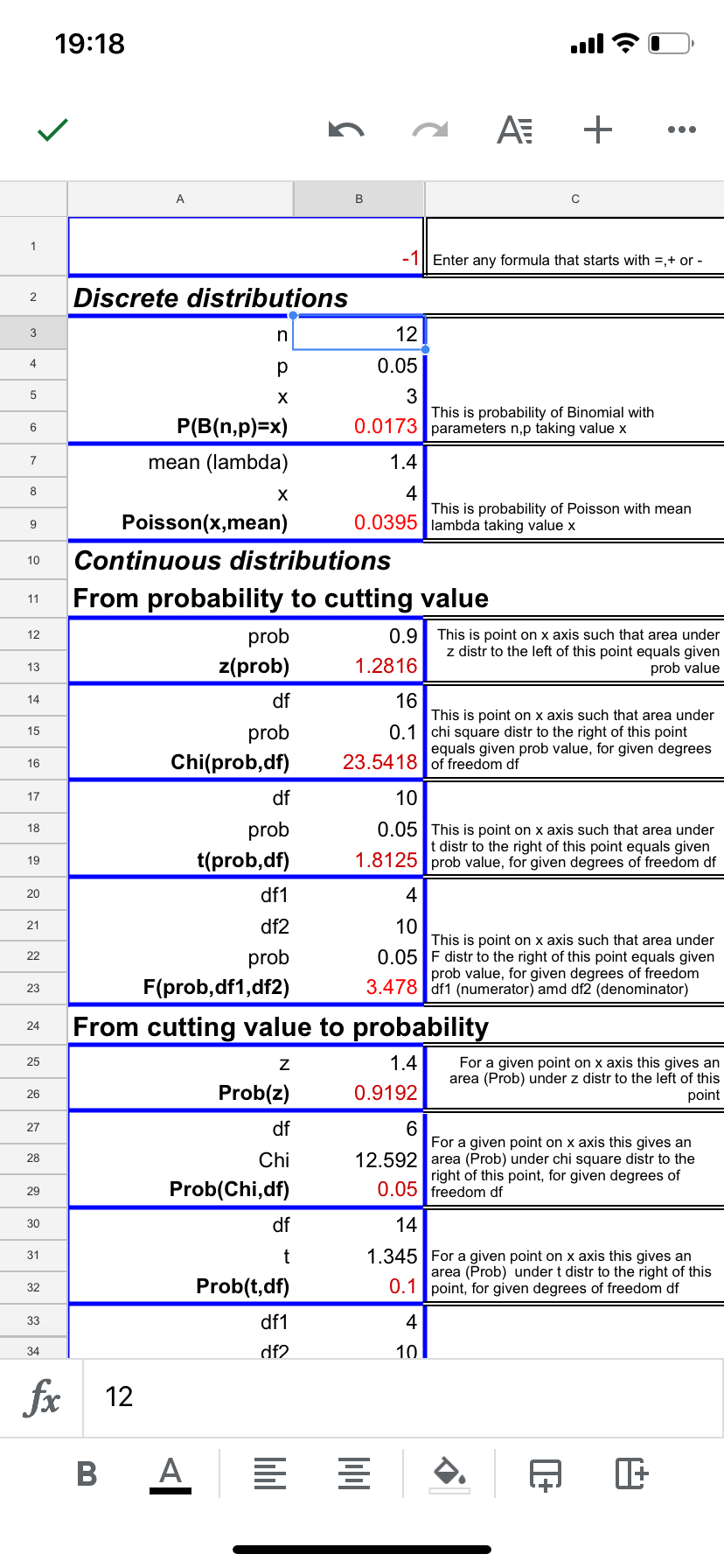2
Mar 20

## Statistical calculator## Statistical calculator

In my book I explained how one can use Excel to do statistical simulations and replace statistical tables commonly used in statistics courses. Here I go one step further by providing a free statistical calculator that replaces the following tables from the book by Newbold et al.:

Table 1 Cumulative Distribution Function, F(z), of the Standard Normal Distribution Table

Table 2 Probability Function of the Binomial Distribution

Table 5 Individual Poisson Probabilities

Table 7a Upper Critical Values of Chi-Square Distribution with$\nu$ Degrees of Freedom

Table 8 Upper Critical Values of Student’s t Distribution with$\nu$ Degrees of Freedom

Tables 9a, 9b Upper Critical Values of the F Distribution

The calculator is just a Google sheet with statistical functions, see Picture 1:Picture 1. Calculator using Google sheet

## How to use Calculator

1. Open an account at gmail.com, if you haven't already. Open Google Drive.